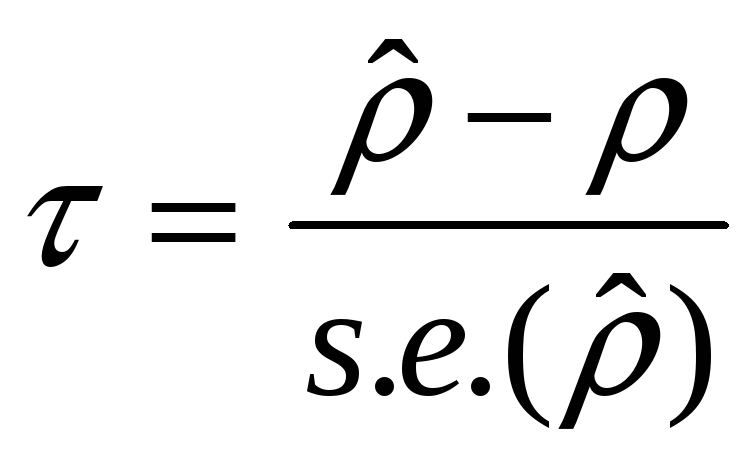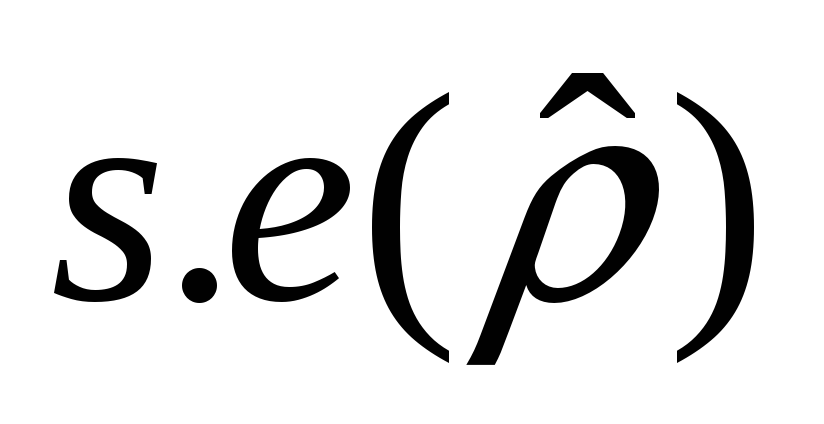# Lecture 23 The Dickey-Fuller Test We have seen that

 Lecture 23 – The Dickey-Fuller Test We have seen that the dynamic behavior of I(1) processes is quite different from the behavior of I(0) processes the way we go about defining and estimating the trend and cyclical components of a time series may depend on whether we assume the series is (trend) stationary or difference stationary. regressions with difference stationary variables need special care. For these reasons we might be interested in testing the null hypothesis of a unit root against the stationary or trend-stationary alternative. Consider the following AR(1) model for yt: yt = ρyt-1 + εt , εt ~ iid (0,σ2) -1 < ρ < 1 If ρ < 1, yt ~ I(0), mean 0 and var σ2/(1-ρ2) If ρ = 1, yt ~ I(1), a random walk The OLS estimator of ρ is consistent for all ρ; it is super-consistent when ρ = 1. The OLS t-statisticwhereis the OLS s.e. of ρ-hat, is asymptotically standard normal whenDownload 274.11 Kb.Share with your friends: